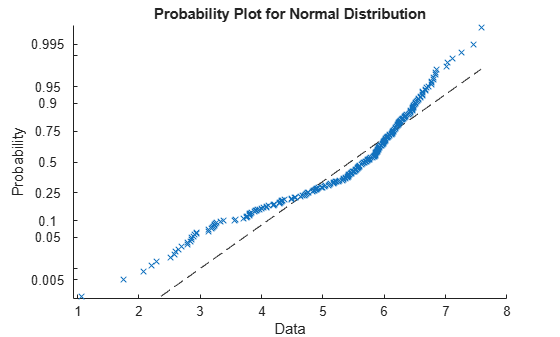Exploratory Analysis of Data

This example shows how to explore the distribution of data using descriptive statistics.

Generate sample data.

Generate a vector containing randomly-generated sample data.

rng default  % For reproducibility
x = [normrnd(4,1,1,100),normrnd(6,0.5,1,200)];

Plot a histogram.

Plot a histogram of the sample data with a normal density fit. This provides a visual comparison of the sample data and a normal distribution fitted to the data.

histfit(x)The distribution of the data appears to be left skewed. A normal distribution does not look like a good fit for this sample data.

Obtain a normal probability plot.

Obtain a normal probability plot. This plot provides another way to visually compare the sample data to a normal distribution fitted to the data.

probplot('normal',x)The probability plot also shows the deviation of data from normality.

Compute the quantiles.

Compute the quantiles of the sample data.

p = 0:0.25:1;
y = quantile(x,p);
z = [p;y]
z = 2×5

0    0.2500    0.5000    0.7500    1.0000
1.0557    4.7375    5.6872    6.1526    7.5784

Create a box plot to visualize the statistics.

boxplot(x)The box plot shows the 0.25, 0.5, and 0.75 quantiles. The long lower tail and plus signs show the lack of symmetry in the sample data values.

Compute descriptive statistics.

Compute the mean and median of the data.

y = [mean(x),median(x)]
y = 1×2

5.3438    5.6872

The mean and median values seem close to each other, but a mean smaller than the median usually indicates that the data is left skewed.

Compute the skewness and kurtosis of the data.

y = [skewness(x),kurtosis(x)]
y = 1×2

-1.0417    3.5895

A negative skewness value means the data is left skewed. The data has a larger peakedness than a normal distribution because the kurtosis value is greater than 3.

Compute z-scores.

Identify possible outliers by computing the z-scores and finding the values that are greater than 3 or less than -3.

Z = zscore(x);
find(abs(Z)>3);

Based on the z-scores, the 3rd and 35th observations might be outliers.